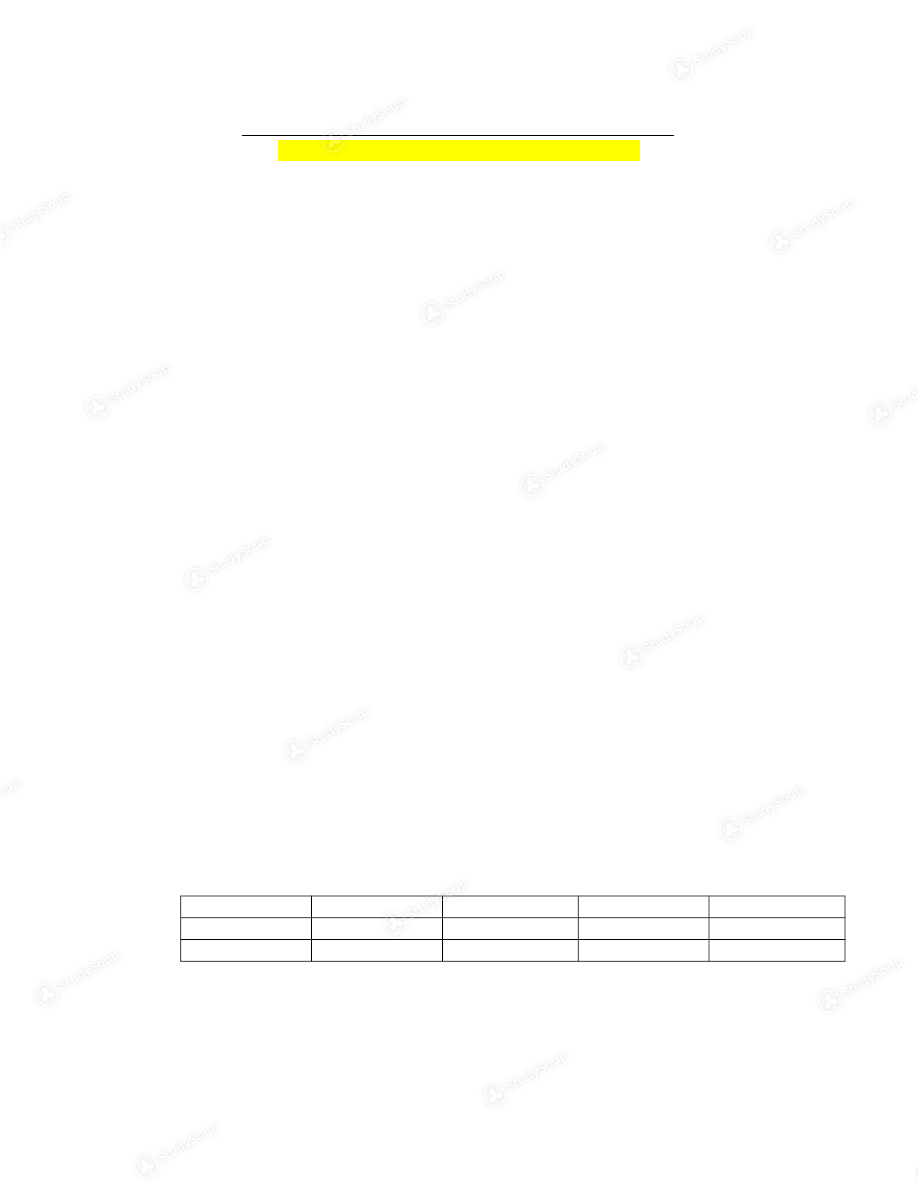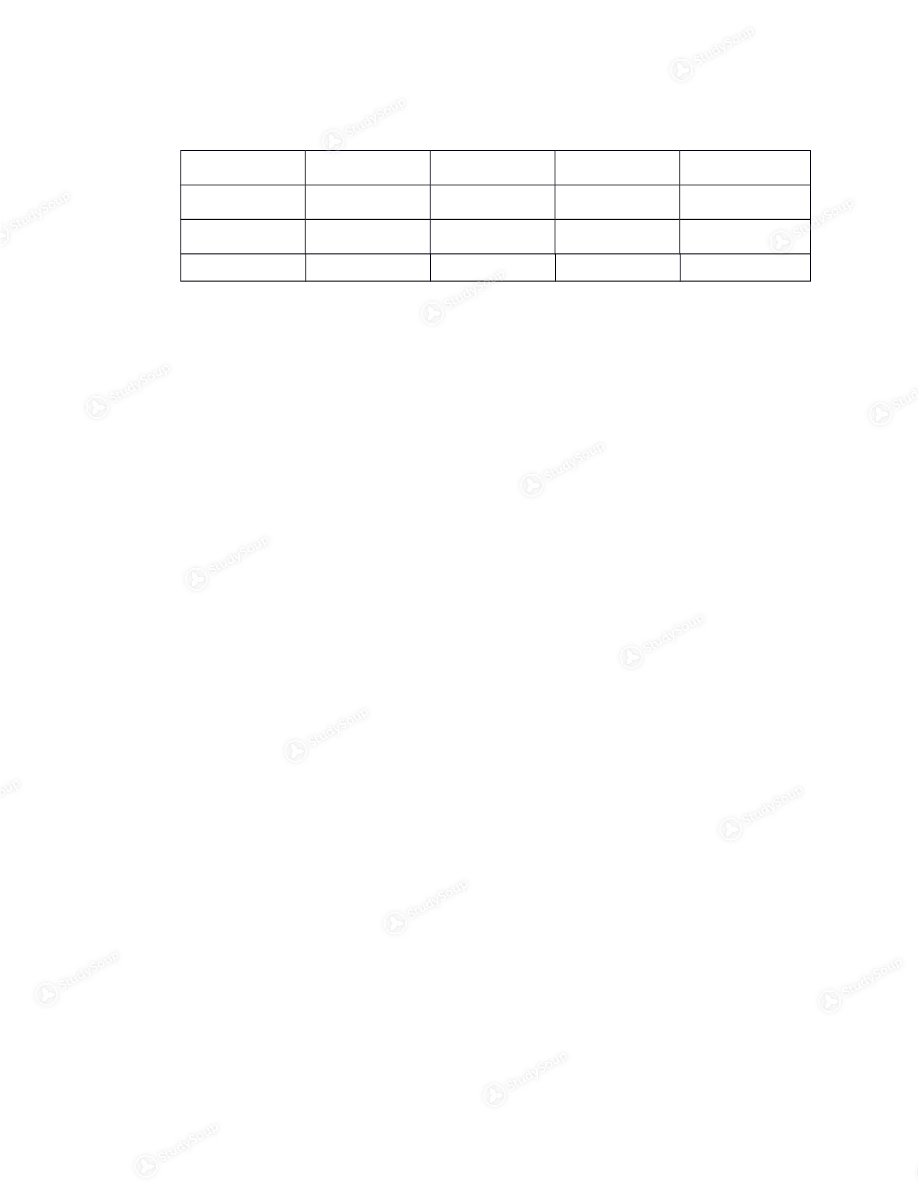×

# UA - STAT 111 - Class Notes - Week 23

### Created by: Carter Cox Elite Notetaker

> > > > UA - STAT 111 - Class Notes - Week 23

UA - STAT 111 - Class Notes - Week 23

##### Description: Covers all formulas that will be on the exam as well as formulas that might show up on certain exam, depending on the color
0 5 3 4 Reviews
This preview shows pages 1 - 2 of a 3 page document. to view the rest of the contentEC-111 Final Exam Formulas (Some formulas will not be on very test) Equilibrium - (Quantity Demanded = Quantity Supplied) Surplus - Above equilibrium
- QS is greater than QD
Shortage - Below equilibrium
- QD is greater than QS
Elasticity of Demand - (% Change in Quantity Demanded)/ (% Change in Price)
- Midpoint
o (End – Start)/ Start
o (New – Old)/ Old
Revenue  - (Price X Quantity) GDP (Total Spending) - (Y = C + I + G + NX)
- C = Consumption
- I = Investment
- G = Government Purchases
- NX = Net Exports
o NX = Exports – Imports GDP Deflator  - (100 X (Nominal GDP/ Real GDP)) Nominal GDP  - (Current Quantity X Current Price) Pizza Pizza Latte Latte Year  Price  Quantity Price  Quantity 2010 \$10 400 \$2 1000 - 2010 o \$10 X 400 + \$2 X 1000 = 6000Real GDP - Base Year (Given) X Current Quantity  Pizza Pizza Latte  Latte Year Price Quantity Price  Quantity  2010 \$10 400 \$2 1000 2011 \$11 500 \$2.50 1100 - Real GDP for 2011 o \$10 X 500 + \$2 X 1100 = 7200
o 2010 is base year
CPI  - (100 x (Cost of Basket in Current Year/ Cost of Basket in Base  Year) Inflation Rate  - ((CPI this year – CPI Last year)/ CPI last year) x 100 Labor Force  - Total number of workers
- Employed + Unemployed
Unemployment Rate - Percent of the labor force that is unemployed
- (100 x (number of unemployed/ Labor Force)
Labor Force Participation Rate - Percent of the adult population that is in the labor force
- (100 X (Labor Force/ Adult Participation)
Population - Labor Force + Not in labor force  Loanable Funds - 100 x (Labor Force/ Population)  Trade Deficit  - Excess of imports over exports
- NX less than 0 and Y is less than C + I + G
Trade Surplus  - Excess of exports over imports
- NX is greater than 0 and Y is greater than C + I + G

This is the end of the preview. Please to view the rest of the contentJoin more than 18,000+ college students at University of Alabama - Tuscaloosa who use StudySoup to get ahead
3 Pages 34 Views 27 Unlocks
• Notes, Study Guides, Flashcards + More!Join more than 18,000+ college students at University of Alabama - Tuscaloosa who use StudySoup to get ahead